Courses

20 Questions MCQ Test Mathematics Olympiad Class 7 | Olympiad Test: Decimals -1

Description
Attempt Olympiad Test: Decimals -1 | 20 questions in 40 minutes | Mock test for Class 7 preparation | Free important questions MCQ to study Mathematics Olympiad Class 7 for Class 7 Exam | Download free PDF with solutions
QUESTION: 1

How many pieces of plywood each 0.35 cm thick are required to make a pile 1.89 m high?

Solution:

Number of pieces of plywood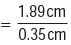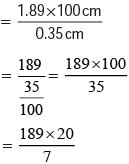= 27 × 20 = 540

QUESTION: 2

A car covers a distance of 31.8 km in 2.4 litres of petrol. How much distance will it cover in 5 litres of petrol ?

Solution:

Distance covered in litres of petrol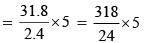= 66.25 km

QUESTION: 3

Each side of a polygon is 3.9 cm and its perimeter is 31.2 cm. How many sides does the polygon have?

Solution:

Let the polygon has n sides.
3.9 × n = 31.2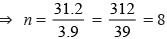QUESTION: 4

The weight of 47 bags of sugar is 3889.25 kg. What is the weight of each bag ?

Solution:

Weight of each bag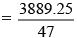= 82.75 kg

QUESTION: 5

0.4 × 0.7 × 0.4 = ?

Solution:

0.4 × 0.7 × 0.4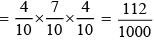= 0.112

QUESTION: 6

0.4 ÷0.4 ÷0.4 = ?

Solution:

0.4 ÷ 0.4 ÷ 0.4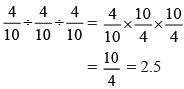QUESTION: 7

Mukesh bought 17.5 litres of Mustard oil for Rs 1550.50. What is the cost per litre?

Solution: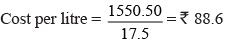QUESTION: 8

The product of two decimals is 2.1033. If one of them is 3.69, what is the other?

Solution: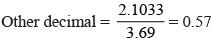QUESTION: 9

1 metre of cloth costs Rs 109.25. What is the cost of 19.5 metres of this cloth?

Solution:

Total cost = 109.25 × 19.5
=  Rs 2130.375

QUESTION: 10

A bus can cover 31.25 km in half an hour. How much distance it cover in 16 hours ?

Solution:

Distance = speed × Time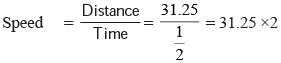= 62.5
km Distance = 62.5 ×16 = 1000 km

QUESTION: 11

A small bottle hold 0.645 kg of juice. How much juice will be there in 68 such bottles?

Solution:

68 × 0.645 = 43.86 kg

QUESTION: 12

(0.25)2 – (0.19)2 = ?

Solution:

(0.25)2 – (019)2
= 0.0625 – 0.0361
= 0.0264

QUESTION: 13

What is the product of 11.1 × 1.1 × 0.11 ?

Solution:

11.1×1.1 × 0.11 = 1.3431

QUESTION: 14

Mohan purchased a notebook for Rs 27.75, a pencil for Rs 4.25 and a Pen 26.45. He gives a 100– rupee note to the shopkeeper. What amount did he get back ?

Solution:

100 – (27.75 + 4.25 + 26 .45)
= 100–58.45 = 41.55

QUESTION: 15

Find the sum of 67.66, 6.766, 676.6 & 0.676.

Solution:

67.66 + 6.766 + 676.6 +0.676 = 751.702

QUESTION: 16

Find the simplified value of
79.1 – 27.73 + 18.07 – 46.37.

Solution:

(79.1+18.07) –(27.73 + 46.37)
= 97.17 –74.1 = 23.07

QUESTION: 17

Naresh bought 4 kg 270 g potato, 2 kg 170 g onion and some tomato. If the total weight of these vegetables is 11 kg 230 g. What is the weight of tomato?

Solution:

Weight of tomato
= 11.230 – (4.270 + 2.170)
= 11.23 –6.44 = 4.79 kg

QUESTION: 18

What is the correct ascending order?

Solution:
QUESTION: 19

45 mm = ? km

Solution: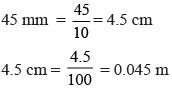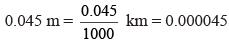QUESTION: 20

What is the product of 0.9 × 0.7 × 6.3?

Solution:

0.9 × 0.7 × 6.3
= 0.63 × 6.3 = 3.969Use Code STAYHOME200 and get INR 200 additional OFF Use Coupon Code

Track your progress, build streaks, highlight & save important lessons and more!

Similar ContentRelated tests